# RS Aggarwal Class 10 Solutions Chapter 11 Arithmetic Progressions MCQS

## RS Aggarwal Class 10 Solutions Chapter 11 Arithmetic Progressions MCQS

These Solutions are part of RS Aggarwal Solutions Class 10. Here we have given RS Aggarwal Solutions Class 10 Chapter 11 Arithmetic Progressions MCQS

Other Exercises

Choose the correct answer in each of the following questions.
Question 1.
Solution: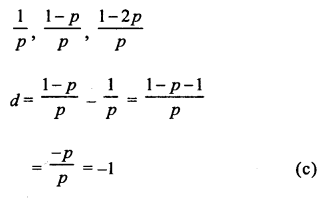Question 2.
Solution: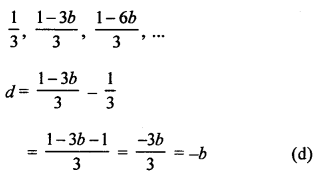Question 3.
Solution: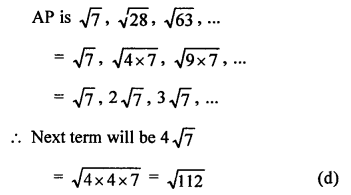Question 4.
Solution: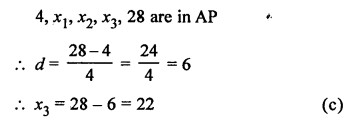Question 5.
Solution: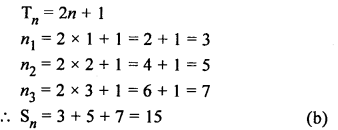Question 6.
Solution: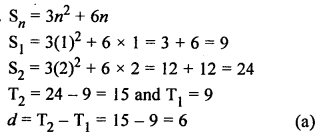Question 7.
Solution: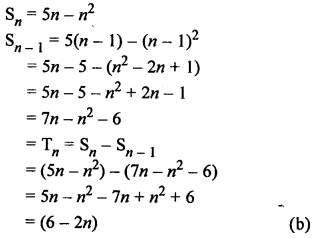Question 8.
Solution: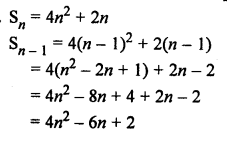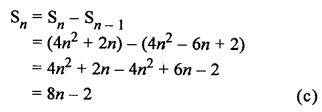Question 9.
Solution: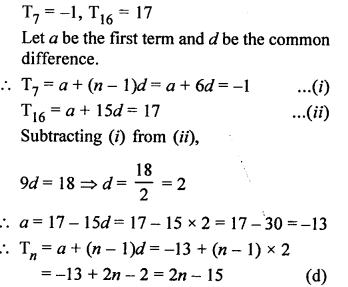Question 10.
Solution: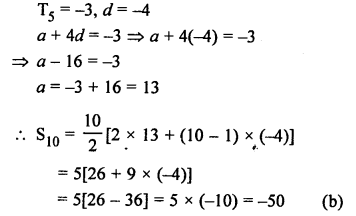Question 11.
Solution: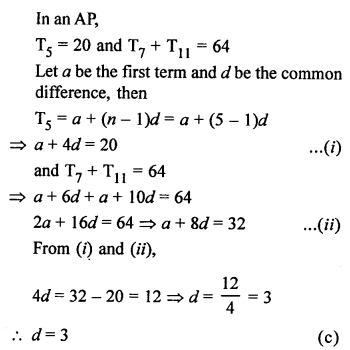Question 12.
Solution: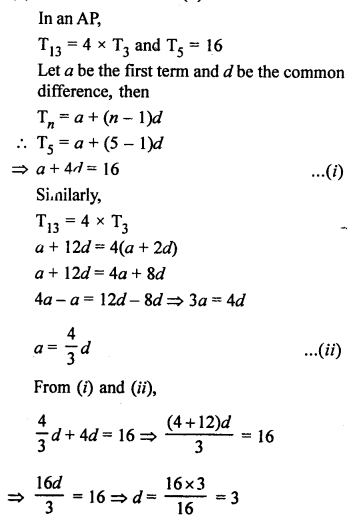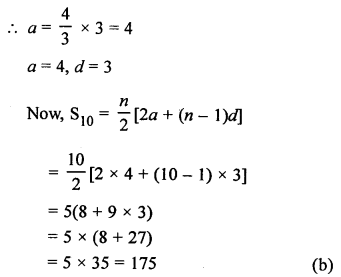Question 13.
Solution: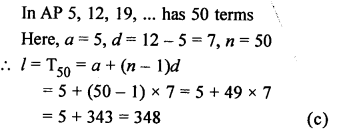Question 14.
Solution:
Sum of first n odd natural numbers = (n)²
Sum of first 20 odd natural numbers = (20)² = 400 (c)

Question 15.
Solution:
First 40 positive integers divisible by 6 are 6, 12, 18, 24, … to 40 terms
Here, a = 6, d = 6, n = 40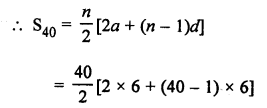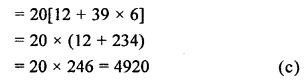Question 16.
Solution: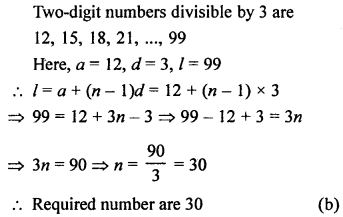Question 17.
Solution: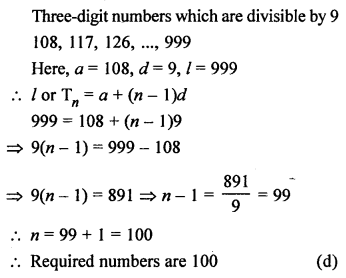Question 18.
Solution:
In an AP,
a18 – a14 = 32
Let a be the first term and d be the common difference, then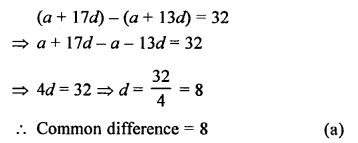Question 19.
Solution: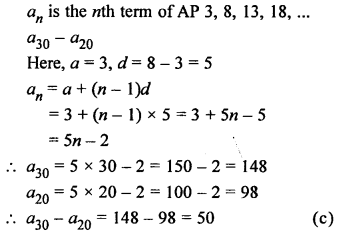Question 20.
Solution: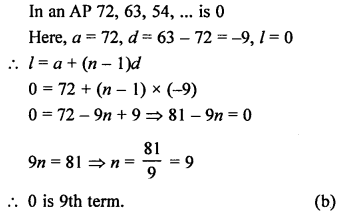Question 21.
Solution: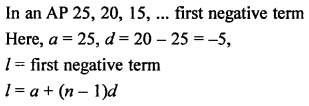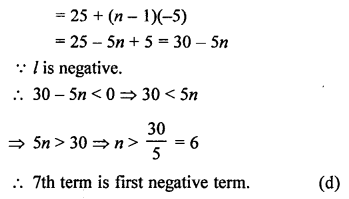Question 22.
Solution: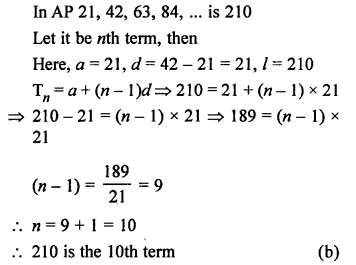Question 23.
Solution: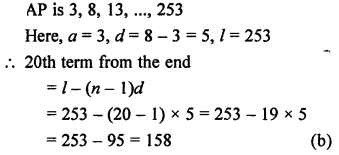Question 24.
Solution: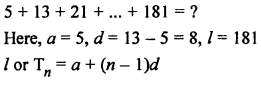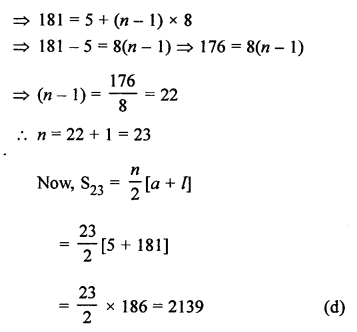Question 25.
Solution:
Sum of first 16 terms of AP 10, 6, 2, …
Here, a = 10, d = 6 – 10 = -4, n = 16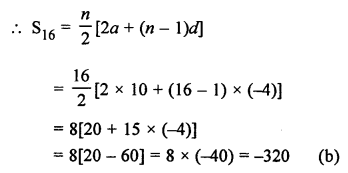Question 26.
Solution: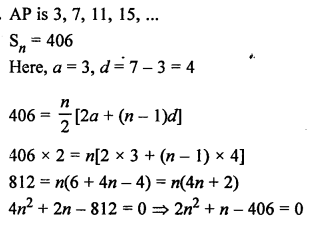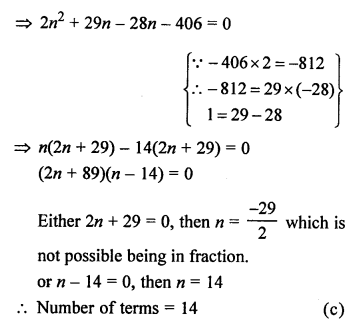Question 27.
Solution: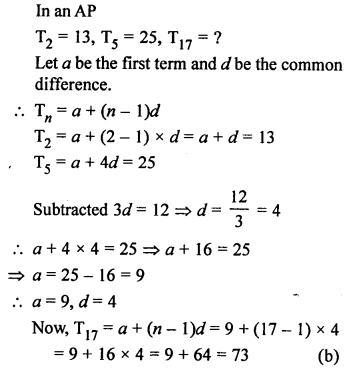Question 28.
Solution:
In an AP, T17 = T10 + 21
Let a be the first term and d be the common difference, then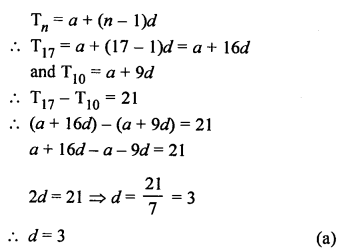Question 29.
Solution: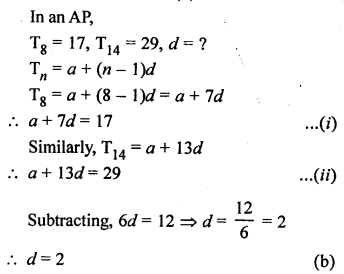Question 30.
Solution: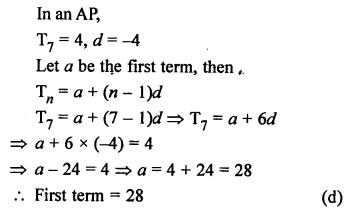Hope given RS Aggarwal Solutions Class 10 Chapter 11 Arithmetic Progressions MCQS are helpful to complete your math homework.

If you have any doubts, please comment below. Learn Insta try to provide online math tutoring for you.